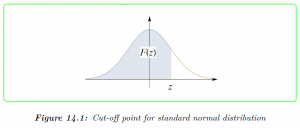9
Dec 16

## Ditch statistical tables if you have a computer

You don't need statistical tables if you have Excel or Mathematica. Here I give the relevant Excel and Mathematica functions described in Chapter 14 of my book. You can save all the formulas in one spreadsheet or notebook and use it multiple times.

###For a given real$z$, the value of the distribution function of the standard normal is$F(z)=\frac{1}{\sqrt{2\pi }}\int_{-\infty }^{z}\exp (-t^{2}/2)dt.$

In Excel, use the formula =NORM.S.DIST(z,TRUE).

In Mathematica, enter CDF[NormalDistribution[0,1],z]

### Probability Function of the Binomial Distribution

For given number of successes$x,$ number of trials$n$ and probability$p$ the probability is$P(Binomial=x)=C_{x}^{n}p^{x}(1-p)^{n-x}$.

In Excel, use the formula =BINOM.DIST(x,n,p,FALSE)

In Mathematica, enter PDF[BinomialDistribution[n,p],x]

### Cumulative Binomial Probabilities

For a given cut-off value$x,$ number of trials$n$ and probability$p$ the cumulative probability is$P(Binomial\leq x)=\sum_{t=0}^{x}C_{t}^{n}p^{t}(1-p)^{n-t}.$
In Excel, use the formula =BINOM.DIST(x,n,p,TRUE).

In Mathematica, enter CDF[BinomialDistribution[n,p],x]

### Values of the exponential function$e^{-\lambda}$$e^{-\lambda}$

In Excel, use the formula =EXP(-lambda)

In Mathematica, enter Exp[-lambda]

### Individual Poisson Probabilities

For given number of successes$x$ and arrival rate$\lambda$ the probability is$P(Poisson=x)=\frac{e^{-\lambda }\lambda^{x}}{x!}.$
In Excel, use the formula =POISSON.DIST(x,lambda,FALSE)

In Mathematica, enter PDF[PoissonDistribution[lambda],x]

### Cumulative Poisson Probabilities

For given cut-off$x$ and arrival rate$\lambda$ the cumulative probability is$P(Poisson\leq x)=\sum_{t=0}^{x}\frac{e^{-\lambda }\lambda ^{t}}{t!}.$
In Excel, use the formula =POISSON.DIST(x,lambda,TRUE)

In Mathematica, enter CDF[PoissonDistribution[lambda],x]

### Cutoff Points of the Chi-Square Distribution Function

######For given probability of the right tail$\alpha$ and degrees of freedom$\nu$, the cut-off value (critical value)$\chi _{\nu,\alpha }^{2}$ is a solution of the equation$P(\chi _{\nu}^{2}>\chi _{\nu,\alpha }^{2})=\alpha .$
In Excel, use the formula =CHISQ.INV.RT(alpha,v)

In Mathematica, enter InverseCDF[ChiSquareDistribution[v],1-alpha]

### Cutoff Points for the Student’s t Distribution

######For given probability of the right tail$\alpha$ and degrees of freedom$\nu$, the cut-off value$t_{\nu,\alpha }$ is a solution of the equation$P(t_{\nu}>t_{\nu,\alpha })=\alpha$.
In Excel, use the formula =T.INV(1-alpha,v)

In Mathematica, enter InverseCDF[StudentTDistribution[v],1-alpha]

### Cutoff Points for the F Distribution

######For given probability of the right tail$\alpha$, degrees of freedom$v_1$ (numerator) and$v_2$ (denominator), the cut-off value$F_{v_1,v_2,\alpha }$ is a solution of the equation$P(F_{v_1,v_2}>F_{v_1,v_2,\alpha })=\alpha$.

In Excel, use the formula =F.INV.RT(alpha,v1,v2)

In Mathematica, enter InverseCDF[FRatioDistribution[v1,v2],1-alpha]